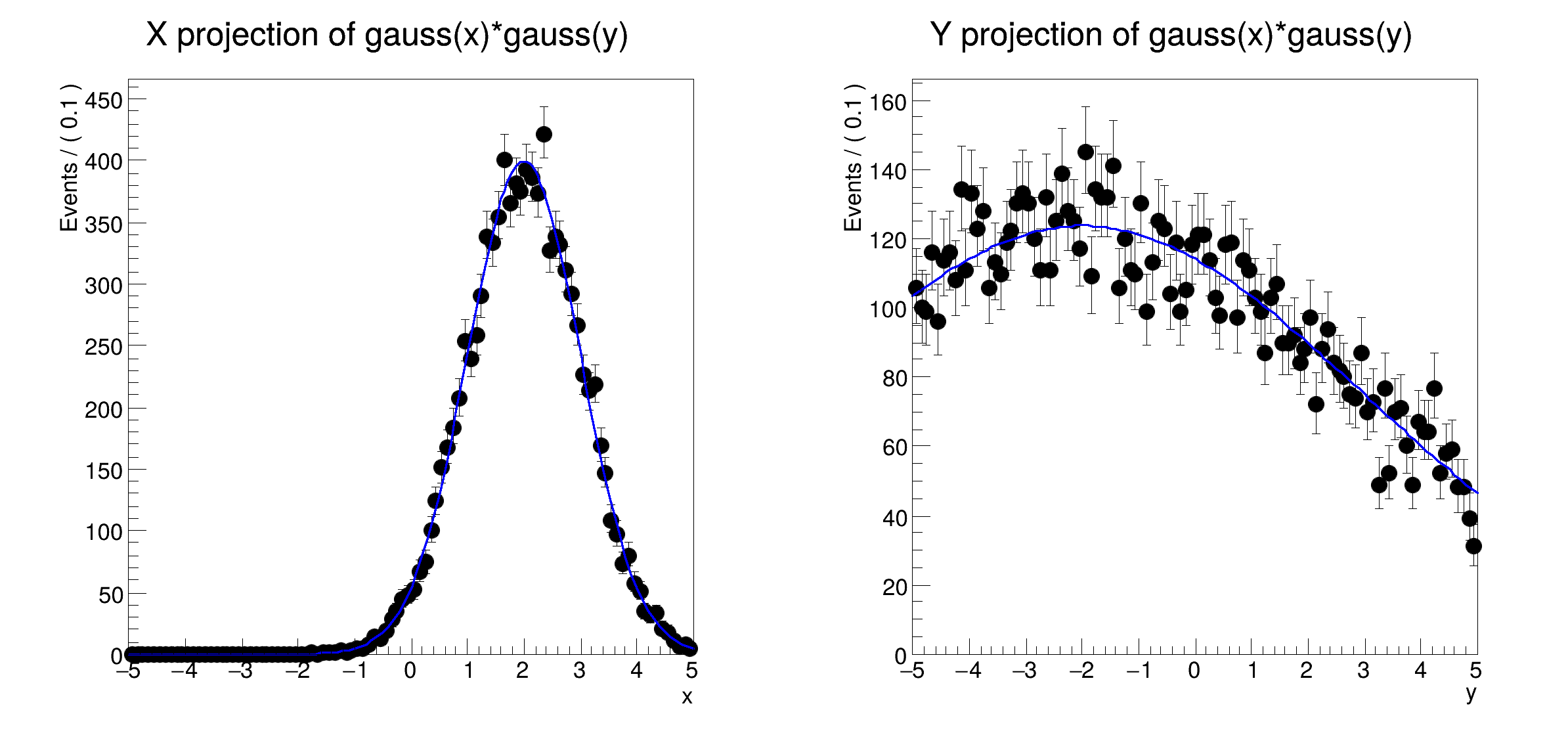ROOT   Reference Guiderf304_uncorrprod.C File Reference

## Detailed DescriptionMultidimensional models: simple uncorrelated multi-dimensional pdfs

pdf = gauss(x,mx,sx) * gauss(y,my,sy)[#0] WARNING:InputArguments -- The parameter 'sigmax' with range [-inf, inf] of the RooGaussian 'gaussx' exceeds the safe range of (0, inf). Advise to limit its range.
[#0] WARNING:InputArguments -- The parameter 'sigmay' with range [-inf, inf] of the RooGaussian 'gaussy' exceeds the safe range of (0, inf). Advise to limit its range.
[#1] INFO:Plotting -- RooAbsReal::plotOn(gaussxy) plot on x integrates over variables (y)
[#1] INFO:Plotting -- RooAbsReal::plotOn(gaussxy) plot on y integrates over variables (x)
#include "RooRealVar.h"
#include "RooDataSet.h"
#include "RooGaussian.h"
#include "RooProdPdf.h"
#include "TCanvas.h"
#include "TAxis.h"
#include "RooPlot.h"
using namespace RooFit;
{
// C r e a t e c o m p o n e n t p d f s i n x a n d y
// ----------------------------------------------------------------
// Create two pdfs gaussx(x,meanx,sigmax) gaussy(y,meany,sigmay) and its variables
RooRealVar x("x", "x", -5, 5);
RooRealVar y("y", "y", -5, 5);
RooRealVar meanx("mean1", "mean of gaussian x", 2);
RooRealVar meany("mean2", "mean of gaussian y", -2);
RooRealVar sigmax("sigmax", "width of gaussian x", 1);
RooRealVar sigmay("sigmay", "width of gaussian y", 5);
RooGaussian gaussx("gaussx", "gaussian PDF", x, meanx, sigmax);
RooGaussian gaussy("gaussy", "gaussian PDF", y, meany, sigmay);
// C o n s t r u c t u n c o r r e l a t e d p r o d u c t p d f
// -------------------------------------------------------------------
// Multiply gaussx and gaussy into a two-dimensional pdf gaussxy
RooProdPdf gaussxy("gaussxy", "gaussx*gaussy", RooArgList(gaussx, gaussy));
// S a m p l e p d f , p l o t p r o j e c t i o n o n x a n d y
// ---------------------------------------------------------------------------
// Generate 10000 events in x and y from gaussxy
RooDataSet *data = gaussxy.generate(RooArgSet(x, y), 10000);
// Plot x distribution of data and projection of gaussxy on x = Int(dy) gaussxy(x,y)
RooPlot *xframe = x.frame(Title("X projection of gauss(x)*gauss(y)"));
data->plotOn(xframe);
gaussxy.plotOn(xframe);
// Plot x distribution of data and projection of gaussxy on y = Int(dx) gaussxy(x,y)
RooPlot *yframe = y.frame(Title("Y projection of gauss(x)*gauss(y)"));
data->plotOn(yframe);
gaussxy.plotOn(yframe);
// Make canvas and draw RooPlots
TCanvas *c = new TCanvas("rf304_uncorrprod", "rf304_uncorrprod", 800, 400);
c->Divide(2);
c->cd(1);
xframe->GetYaxis()->SetTitleOffset(1.4);
xframe->Draw();
c->cd(2);
yframe->GetYaxis()->SetTitleOffset(1.4);
yframe->Draw();
}
#define c(i)
Definition: RSha256.hxx:101
Option_t Option_t TPoint TPoint const char GetTextMagnitude GetFillStyle GetLineColor GetLineWidth GetMarkerStyle GetTextAlign GetTextColor GetTextSize void data
RooArgList is a container object that can hold multiple RooAbsArg objects.
Definition: RooArgList.h:22
RooArgSet is a container object that can hold multiple RooAbsArg objects.
Definition: RooArgSet.h:56
RooDataSet is a container class to hold unbinned data.
Definition: RooDataSet.h:57
Plain Gaussian p.d.f.
Definition: RooGaussian.h:24
A RooPlot is a plot frame and a container for graphics objects within that frame.
Definition: RooPlot.h:43
TAxis * GetYaxis() const
Definition: RooPlot.cxx:1279
void Draw(Option_t *options=nullptr) override
Draw this plot and all of the elements it contains.
Definition: RooPlot.cxx:652
RooProdPdf is an efficient implementation of a product of PDFs of the form.
Definition: RooProdPdf.h:33
RooRealVar represents a variable that can be changed from the outside.
Definition: RooRealVar.h:40
virtual void SetTitleOffset(Float_t offset=1)
Set distance between the axis and the axis title.
Definition: TAttAxis.cxx:298
The Canvas class.
Definition: TCanvas.h:23
Double_t y[n]
Definition: legend1.C:17
Double_t x[n]
Definition: legend1.C:17
The namespace RooFit contains mostly switches that change the behaviour of functions of PDFs (or othe...
Definition: Common.h:18
const char * Title
Definition: TXMLSetup.cxx:68
Date
July 2008

Definition in file rf304_uncorrprod.C.